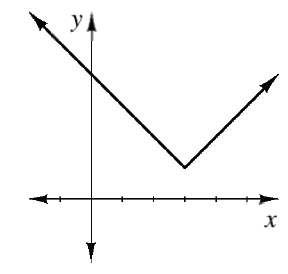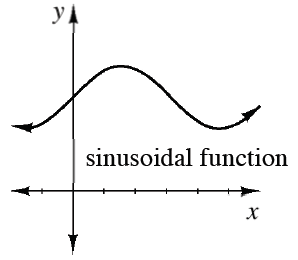### Home > APCALC > Chapter 4 > Lesson 4.1.2 > Problem4-23

4-23.

For parts (a) and (b) below, trace $f\left(x\right)$ on your paper. Then, using a different color, sketch the graph of $y = f^\prime\left(x\right)$ for the function given.

1.• $f\left(x\right)$ has a cusp at $x = 3$, so $x ^\prime\left(x\right)$ should have a jump at $x = 3$.

1.• The max and min on a smooth continuous graph are the location of zeros on the derivative graph.